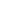# Mathematics in the Ancient World

Ancient Babylonians, writing with wooden sticks on clay, developed a complicated sexagesimal numeral system which allowed them to perform addition, multiplication and division. Ancient Egyptians operated with fractions, knew geometric series and the “Egyptian triangle” that later became known as Pythagoras theorem. Ancient Greeks inherited mathematical knowledge from Egyptian and Babylonians, but contrary to them, Greeks asked questions and searched for proofs, developing mathematics into deductive science with definitions, postulates, and theorems. Despite all difficulties, some women of the ancient time: Enheduanna of Mesopotamia, Theano of Crotone, Aglaonike of Thessaly, Hypatia of Alexandria, demonstrated achievements in mathematics.

#### DELIVERY MODE

• Face-to-Face

• Science Awakening I: Egyptian, Babylonian and Greek Mathematics, B. L. Van Der Waerden. 2005, 4th Edition.
• Women in Maths: From Hypatia to Emmy Noether, Joaquín Navarro. RBA Coleccionables, S.A., 2013.

#### COURSE OUTLINE

• Main chronology of ancient mathematics in Babylon, Egypt and Greece. Numeral systems in the ancient world, operations on numbers and figures. Algebraic and geometric problems. Main mathematical artefacts: Rhind papyrus, Plimpton tablet.
• Greek numeral systems. Zeno’s paradoxes. Three great mathematical problems: doubling the cube; trisecting the angle; squaring the circle. Pythagorean number theory. Irrationality. Elements by Euclid. Archimedes: his mathematical discoveries, legends about him.
• Female mathematicians of the Ancient World: Enheduanna, a Mesopotamian Princess and a high priestess of moon god Nanna; Sumerian scribe Nin-un-il; Athyrta, an Egyptian princess working on astronomy and natural philosophy; Theano of Crotone, wife of Pythagoras and a presumably head of Pythagoras school; Aglaonike of Thessaly, an astronomer predicting moon eclipses; Hypatia of Alexandria, a martyr for science.

#### LEARNING OUTCOMES

By the end of this course, students should be able to:

1. Understand ancient numeral systems.
2. Know Egyptian and Babylonian counting techniques for multiplying, dividing, solving arithmetical problems, and measuring geometrical objects.
3. Be familiar with the main ancient mathematical artefacts: Rhind papyrus, Plimpton tablet.
4. Understand ancient Greek numeral systems.
5. Know the names of the most significant mathematicians: Pythagoras, Euclid, Archimedes and others.
6. Become acquainted with their main achievements.
7. Know the stories of female mathematicians of the Ancients world and their achievements.
\$158 Limited / \$142

<p>Ancient Babylonians, writing with wooden sticks on clay, developed a complicated sexagesimal numeral system which allowed them to perform addition, multiplication and division. Ancient Egyptians

...
17 Feb
Mo Tu We Th Fr Sa SuInterested in this course but can't attend? Please join the waiting list by clicking .